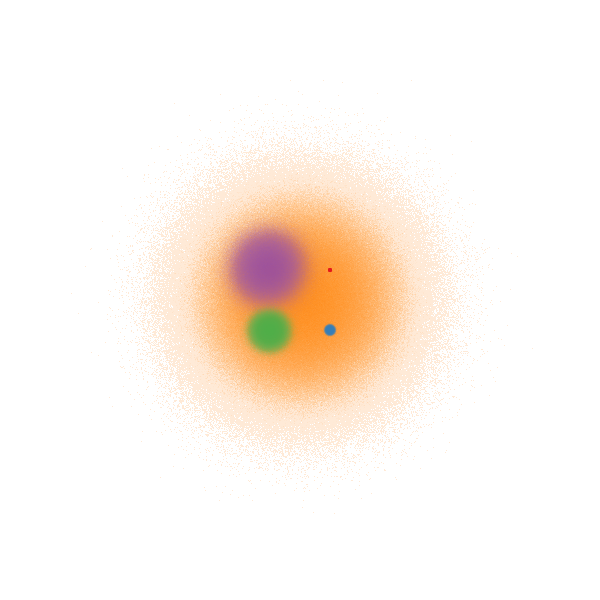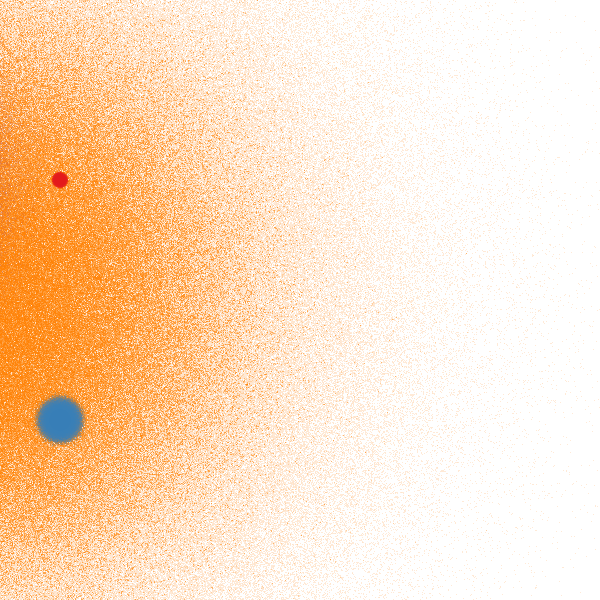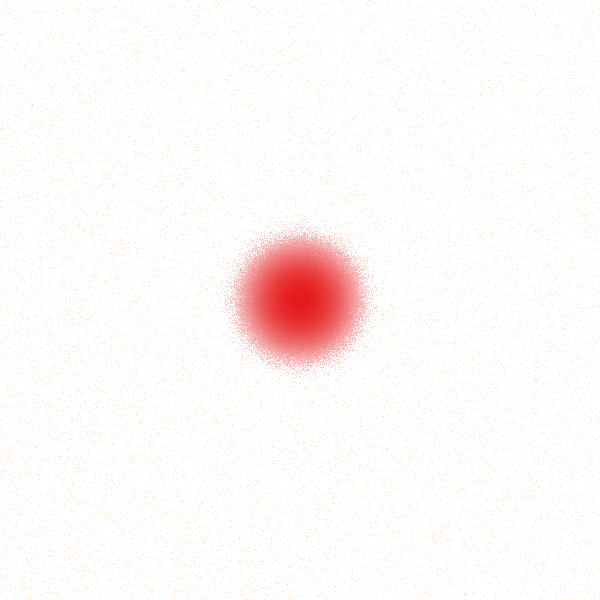# Points¶

This notebook is under construction; please see the pipeline and nyc_taxi notebooks for extensive examples of working with points. For now, this section only includes information about spatially indexed datasets.

# Spatial indexing¶

In most cases, Datashader must iterate through your entire dataset to render any plot, because it cannot assume the datapoints have been sorted in any particular order. Thus, the aggregation performance is dependent on the number of datapoints in your entire dataframe, not just those in the current viewport (x and y range). If you have a large dataset covering a wide area and you want to support fast local operations (e.g. if you have data at a global level but analysis is typically done in small local regions), Datashader supports optionally storing your data in a spatially indexed format. This format makes it very fast to create a new dataframe with only the points from a restricted region, without even needing to bring the other data points into main memory. For a detailed description of the spatial indexing approach used by Datashader, and performance results on a real-world dataset, please see the Spatial Partitioning of Dask DataFrames using Hilbert Curves notebook.

For instance, if you start with an unordered dataframe df of 2 million points:

In :
import pandas as pd, numpy as np, dask.dataframe as dd, datashader as ds
from collections import OrderedDict as odict

num=2000000
np.random.seed(1)

dists = {cat: pd.DataFrame(odict([('x',np.random.normal(x,s,num)),
('y',np.random.normal(y,s,num)),
('val',val),
('cat',cat)]))
for x,  y,  s,  val, cat in
[(  2,  2, 0.03, 10, "d1"),
(  2, -2, 0.10, 20, "d2"),
( -2, -2, 0.50, 30, "d3"),
( -2,  2, 1.00, 40, "d4"),
(  0,  0, 3.00, 50, "d5")] }

df = pd.concat(dists,ignore_index=True)
df["cat"]=df["cat"].astype("category")


You can sort it spatially on x and y using:

In :
import datashader.spatial.points as dsp
%time dsp.to_parquet(df, 'sorted.parq', 'x', 'y', shuffle='disk', npartitions=32)

CPU times: user 24.8 s, sys: 1.1 s, total: 25.9 s
Wall time: 14.4 s


This process involves sorting and then partitioning the entire dataset and then writing the resulting partitions to a Parquet file (which requires the fastparquet library). This is a relatively expensive operation and will take some time, e.g. 5-10 minutes for a 100-million-point dataframe on a 4-core laptop with 16GB of RAM. After this process completes you can load the parquet file as a SpatialPointsFrame, and use it to quickly access subsets of the dataset for a region of interest.

In :
sframe = dsp.read_parquet('sorted.parq')
sframe

Out:
x y val cat
npartitions=32
float64 float64 int64 category[unknown]
... ... ... ...
... ... ... ... ...
... ... ... ...
... ... ... ...

As you can see, the full dataset is split across 32 partitions (this number can be customized using the npartitions argument to the dsp.to_parquet function); the rest of the information is not known because the dataset has not actually been read in yet.

Because SpatialPointsFrame is a subclass of dask.dataframe.DataFrame, you can use sframe anywhere that a Dask DataFrame is accepted. What makes the SpatialPointsFrame class particularly useful, however, is an additional spatial_query method that you can use to request only the subset of partitions that may contain points that overlap with a rectangular query region.

You can use the x_range and y_range properties of the sframe.spatial accessor to check the extents of the entire dataset. These are metadata that were calculated when 'sorted.parq' was created, so accessing this information does not require loading any of the dataset from disk.

In :
print('x_range: ', sframe.spatial.x_range)
print('y_range: ', sframe.spatial.y_range)

x_range:  (-15.216455230786691, 15.514633156049591)
y_range:  (-14.239923338473453, 14.613546446570776)


Here are the range extents of 4 progressively smaller viewports. Notice that the first viewport encompasses the entire dataset.

In :
x_ranges=[(-20, 20), (1, 11), (1.5, 2.5), (2.04, 2.1)]
y_ranges=[(-20, 20), (-5, 5), (1.5, 2.5), (2.04, 2.1)]
ranges = list(zip(x_ranges, y_ranges))


Now, let's define a create_image helper function that inputs the range extents of a viewport, and whether or not to utilize spatial indexing, and performs aggregation and shading. The title of the resulting image includes the number of partitions that were processed and the average aggregation time.

In :
# Read as standard (non-spatial) Dask dataframe

In :
def create_image(x_range, y_range, use_spatial=True):
"""Given a range, time multiple aggregations and average them"""

df = frame if not use_spatial else sframe.spatial_query(x_range, y_range)

canvas = ds.Canvas(x_range=x_range, y_range=y_range)
agg = canvas.points(df,'x','y', agg=ds.count_cat('cat'))
timing = %timeit -n 1 -r 2 -o canvas.points(df,'x','y', agg=ds.count_cat('cat'))
title = "{} partitions, {:.3f} sec".format(df.npartitions, timing.average)



Without spatial indexing, the aggregation time for each of these viewports is nearly constant because the data from all 32 partitions must be processed regardless of how small the viewport is.

In :
tf.Images(*[create_image(x_range, y_range, use_spatial=False) for x_range, y_range in ranges])

551 ms ± 5.98 ms per loop (mean ± std. dev. of 2 runs, 1 loop each)
538 ms ± 3.54 ms per loop (mean ± std. dev. of 2 runs, 1 loop each)
532 ms ± 325 µs per loop (mean ± std. dev. of 2 runs, 1 loop each)
539 ms ± 1.8 ms per loop (mean ± std. dev. of 2 runs, 1 loop each)

Out:
 32 partitions, 0.551 sec32 partitions, 0.538 sec32 partitions, 0.532 sec32 partitions, 0.539 secWith spatial indexing, however, smaller viewports require processing fewer partitions, resulting in significant runtime reductions.

In :
tf.Images(*[create_image(x_range, y_range, use_spatial=True) for x_range, y_range in ranges])

566 ms ± 9.44 ms per loop (mean ± std. dev. of 2 runs, 1 loop each)
288 ms ± 2.33 ms per loop (mean ± std. dev. of 2 runs, 1 loop each)
139 ms ± 1.82 ms per loop (mean ± std. dev. of 2 runs, 1 loop each)
39.2 ms ± 1.45 ms per loop (mean ± std. dev. of 2 runs, 1 loop each)

Out:
 32 partitions, 0.566 sec16 partitions, 0.288 sec